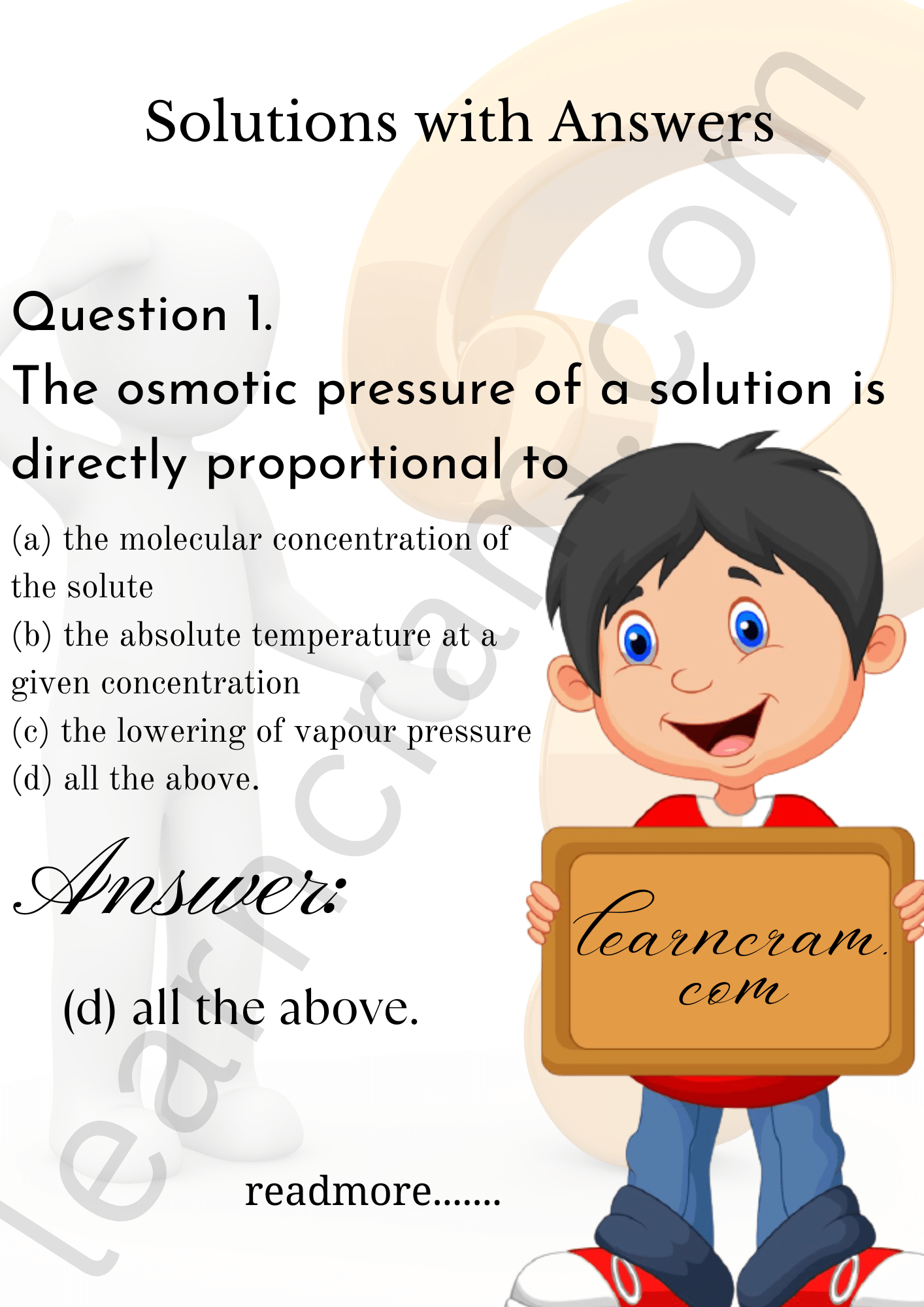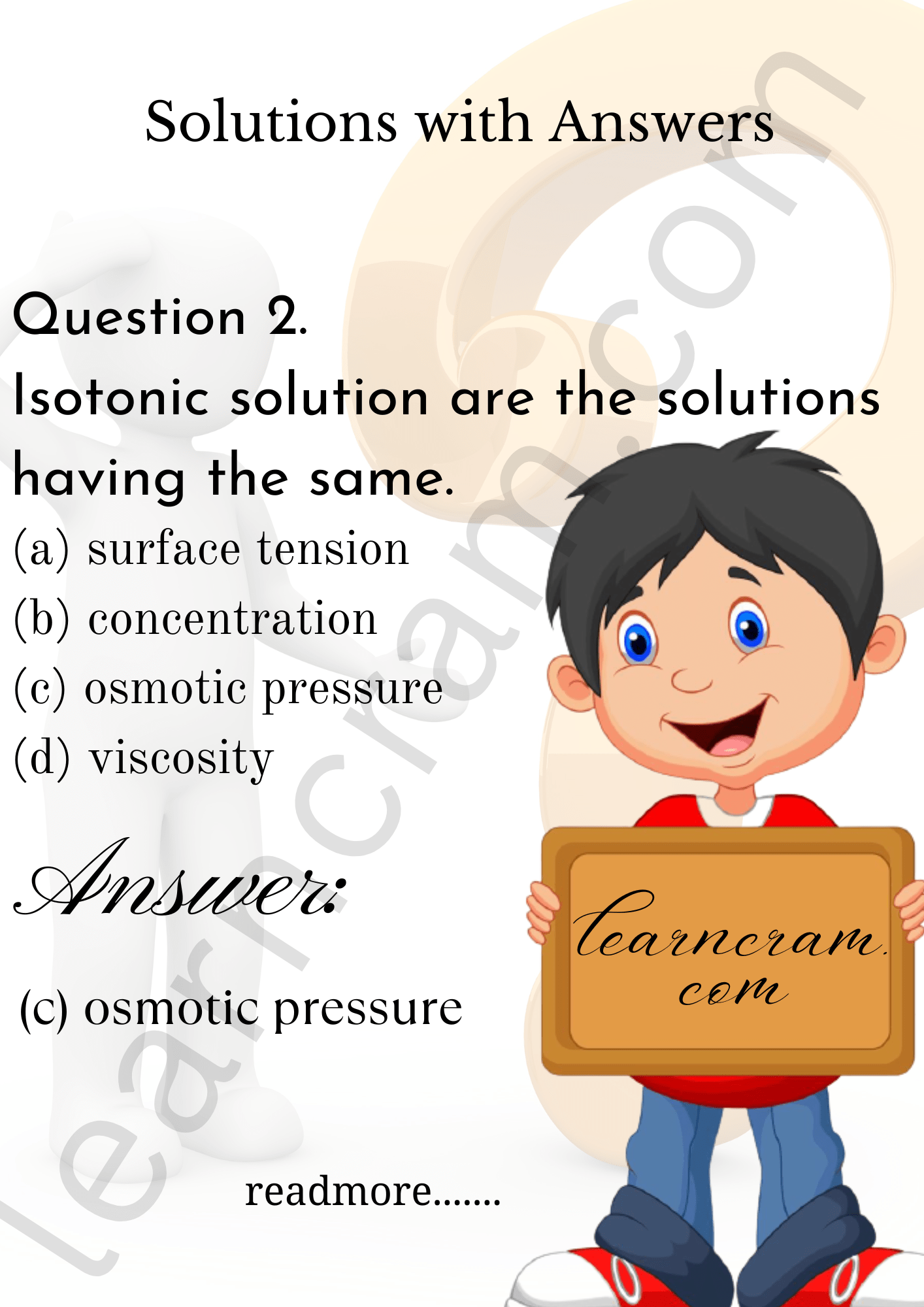# MCQ Questions for Class 12 Chemistry Chapter 2 Solutions with Answers

We have compiled the NCERT MCQ Questions for Class 12 Chemistry Chapter 2 Solutions with Answers Pdf free download covering the entire syllabus. Practice MCQ Questions for Class 12 Chemistry with Answers on a daily basis and score well in exams. Refer to the Solutions Class 12 MCQs Questions with Answers here along with a detailed explanation. Students can also read NCERT Solutions.

## Solutions Class 12 MCQs Questions with Answers

Question 1.
The osmotic pressure of a solution is directly proportional to
(a) the molecular concentration of the solute
(b) the absolute temperature at a given concentration
(c) the lowering of vapour pressure
(d) all the above.Question 2.
Isotonic solution are the solutions having the same.
(a) surface tension
(b) concentration
(c) osmotic pressure
(d) viscosityQuestion 3.
Which of the following is a colligative property?
(a) osmotic pressure
(b) boiling point
(c) vapour pressure
(d) electrical conductivity

Question 4.
Which of the following solutions have highest freezing point?
(a) 0.1 M NaCl
(b) 0.1 M BaCl2
(c) 0.1 M Al2 (SO4)3
(d) 0.1 M urea.

Question 5.
Which of the following 0.1 M aqueous solutions will have the lowest freezing point?
(a) potassium sulphate
(b) sodium chloride
(c) urea
(d) glucose

Question 6.
The mass of (COOH)2. 2H2O needed to prepare 500 ml of 0.1 molar solution is
(a) 12.6 gm
(b) 6.3 gm
(c) 4.5 gm
(d) 9.0 gm

Question 7.
Which of the following solutions has highest osmotic pressure?
(a) 1 M NaCl
(b) 1 M MgCl2
(c) 1 M urea
(d) 1M glucose.

Question 8.
Which of the following solutions (in water) has highest boiling point?
(a) 1 M NaCl
(b) 1 M MgCl2
(c) 1M Urea
(d) 1 M glucose.

Question 9.
Which of the following aqueous solutions containing 10 g of solute in each case, has highest m.pt?
(a) NaCl solution
(b) KC1 solution
(c) sugar solution
(d) glucose solution.

Question 10.
Equal volumes of 0.1 M AgNO3 and 0.2 M NaCl solutions are mixed. The concentration of NO3 ions in mixture solution will be
(a) 0.1 M
(b) 0.05 M
(c) 0.2 M
(d) 0.15 M

Question 11.
The molal freezing point constant for water is 1.86° CM. The freezing point of 0.1 m NaCl solution is expected to be
(a) 13.6
(b) 68
(c) 34
(d) 136

Question 12.
The solution containing 6.8 g of non-ionic solute in 100 g of water was found to freeze at -0.93°C. If Kf for water is 1.86, the mol. mass of solute is
(a) 13.6
(b) 68
(c) 34
(d) 136.

Question 13.
In case of electrolyte which dissociates in solution the Van’t Hoff’s factor, i is
(a) > 1
(b) < 1
(c) = 1
(d) = 0

Question 14.
Which of the following salt will have same value of Vant Hoff’s factor (i) as that of K4[Fe (CN)6]
(a) Al2(SO4)3
(b) NaCl
(c) Al(NO3)3
(d) Na2SO4

Question 15.
120 g of urea is present in 5 L of solution, the active mass of urea is
(a) 0.2
(b) 0.06
(c) 0.4
(d) 0.8

Question 16.
The law stating that the relative lowering of vapour pressure is equal to the mole fraction of a solute in the solution is known as
(a) Henry’s law
(b) Van’t Hoff, Law
(c) Raoult’s law
(d) Ostwald’s dilution law

Question 17.
Azeotropic mixture of HCl and H2O has
(a) 48% HCl
(b) 22.2% HCl
(c) 36% HCl
(d) 20.2% HCl

Question 18.
Which of the following modes of expressing concentration is independent of temperature?
(a) Molarity
(b) Normality
(c) Formality
(d) Molality

Question 19.
A 500 g tooth paste sample has 0.2 g fluoride concentration. What is the concentration of fluorine in terms of ppm level?
(a) 250
(b) 200
(c) 400
(d) 1000

Question 20.
An aqueous solution freezes out -0.186°C (Kf = 1.86°, kb = 0.512). What is the elevation in boiling point?
(a) 0.186
(b) 0 .512
(c) 0.86
(d) 0.0512

Question 21.
The average osmotic pressure of human bipod is 7.8 bar at 37°C. What is the concentration of an aqueous solution of NaCl that could be used in blood stream?
(a) 0.15 mol L-1
(b) 0.30 mol L-1
(c) 0.60 mol L-1
(d) 0.45 mol L-1

Question 22.
Which has the minimum osmotic pressure?
(a) 200 ml of 2 M NaCl
(b) 200 ml of 1 M glucose
(c) 200 ml of 2 M urea
(d) all have same.

Answer: (b) 200 ml of 1 M glucose

Question 23.
Solution A contains 7 g L-1 MgCl2 and solution B contains 7 g L-1 of NaCl. At room temperature, the osmotic pressure of
(a) solution A is greater than B
(b) both have same osmotic pressure
(c) solution B is greater than A
(d) Can’t determine.

Answer: (c) solution B is greater than A

Question 24.
Equimolar solutions in the same solvent have
(a) Same B.Ptbutdifferent F.Pt
(b) Same F. Pt, but different B.Pts.
(c) Same Boiling and same freezing point
(d) Different boiling and different freezing points.

Answer: (c) Same Boiling and same freezing point

Question 25.
The mole fraction of the solute in one molal aqueous solution is
(a) 0.027
(b) 0.036
(c) 0.018
(d) 0.019

Question 26.
The vapour pressure of the two liquids ‘P’ and ‘Q’ are 80 and 60 torr respectively. The total vapour pressure of the solution obtained by mixing 3 mol of P and 2 mole of Q would be
(a) 68 torr
(b) 140 torr
(c) 72 torr
(d) 20 torr

Question 27.
X is dissolved in water. Maximum boiling point is observed when X is ………… (0.1 M each)
(a) CuSO4
(b) BaCl2
(c) NaCl
(d) Urea

Question 28.
If an aqueous solution of glucose is allowed to freeze, then crystals of which substance will be separated and first?
(a) glucose
(b) water
(c) both of these
(d) none of these

Question 29.
If two substances A and B have P$$_{A}^{0}$$ : P$$_{B}^{0}$$ = 1 : 2 and have mole fraction in solution in solution 1 : 2, then mole fraction of A in vapours will be
(a) 0.33
(b) 0.25
(c) 0.52
(d) 0.2

Question 30.
If a is the clegree of dissociation of Na2SO4 the Van’t Hoff factor (i) used for calculating the molecular mass is
(a) 1 + α
(b) 1 – α
(c) 1 + 2α
(d) 1 – 2α

Question 31.
The elevation in boiling point of a solution of 13.44 g of CuCl2 in 1 kg of water (Given Kb = 0.52 K kg mol-1, M. Wt. of CuCl2 = 134.4)
(a) 0.05
(b) 0.1
(c) 0.16
(d) 0.21

Question 32.
Two solutions of a substance (non-electrolyte) are mixed in the following mariner: 480 mL of 1.5 M of first solution + 520 mL of 1.2 M second solution. Wfctat is the molarity of the final mixture?
(a) 1.20 M
(b) 1.50 M
(c) 1.344 M
(d) 2.70 M